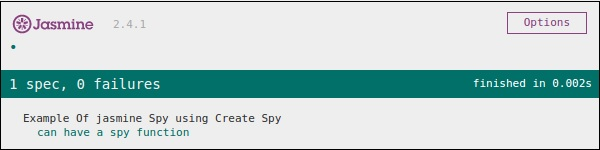# JasmineJS - Overview

Jasmine is an open-source JavaScript framework, capable of testing any kind of JavaScript application. Jasmine follows Behavior Driven Development (BDD) procedure to ensure that each line of JavaScript statement is properly unit tested. By following BDD procedure, Jasmine provides a small syntax to test the smallest unit of the entire application instead of testing it as a whole.

## Why Use Jasmine?

Following are the advantages of using Jasmine over other available JavaScript testing frameworks −

• Jasmine does not depend on any other JavaScript framework.

• Jasmine does not require any DOM.

• All the syntax used in Jasmine framework is clean and obvious.

• Jasmine is heavily influenced by Rspec, JS Spec, and Jspec.

• Jasmine is an open-source framework and easily available in different versions like stand-alone, ruby gem, Node.js, etc.

## How to Use Jasmine?

Jasmine is very easy to implement in any kind of development methodology. All you need to download is the standalone library files from the official website https://jasmine.github.io/ and implement the same in your application.

The detailed environment setup will be described in the next chapter named “Environment setup”. Once you successfully download and unzip the zip file, then you will find the following sub-folders inside that zip file.# JasmineJS - Environment Setup

In this chapter, we will discuss the step-by-step procedure of how to set up a Jasmine based BDD testing application.

Step 1 − Go to the official website of jasmine https://jasmine.github.io/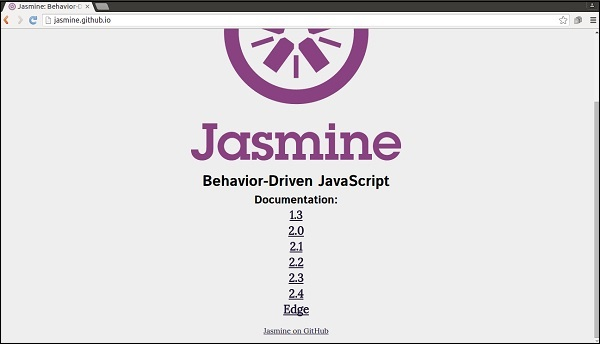Step 2 − Click on any of the version link. It is preferable to use the most recent version that is “Edge”. You will be redirected to the homepage of the selected version.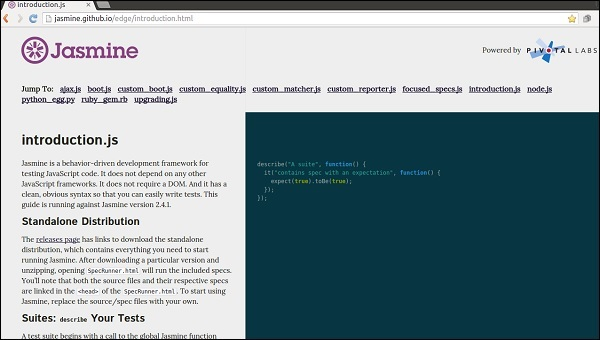Step 3 − Go to the Download section of the homepage and click on the standalone release page.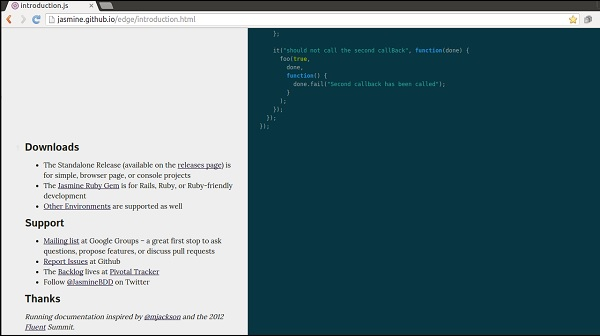Step 4 − Once you are redirected to github release page, download the Zip file from there.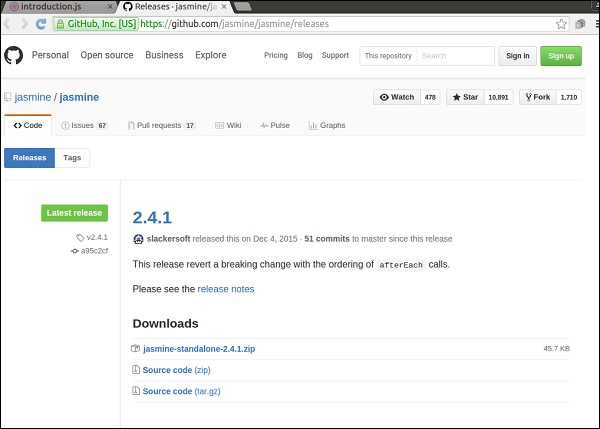Step 5 − Unzip the downloaded jasmine-standalone-2.4.1 folder. You will see the following folder structure.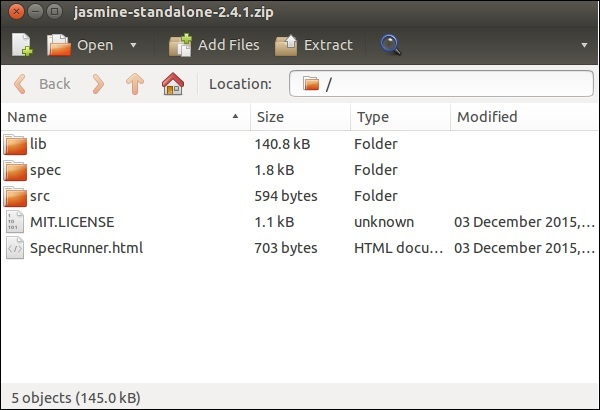Step 6 − Now Create a web application project in your favorite IDE and add this downloaded library files into the application. Here, we have used netbeans IDE. Following is the Directory structure of our application after adding Jasmine framework.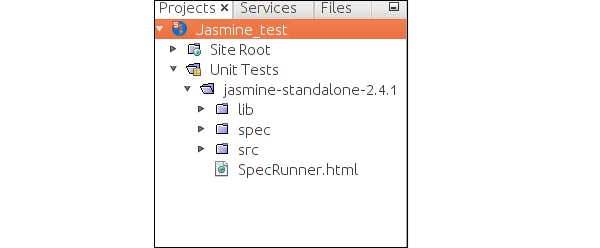Our environment setup is done. Now our application is ready to be tested by Jasmine framework.

# JasmineJS - Writing Text & Execution

In this chapter, we will create a hello world app which will test our “helloworld.js” file. Before developing the hello world app, go back to the previous chapter and make sure that your environment is ready to be tested using Jasmine.

Step 1 − Create a Web application in your IDE

Here we are using NetBeans 8.1 to develop our hello world app in Jasmine. In NetBeans, go to File → New Project → Html5/JS application and create a project. After creating the project, the project directory should look like the following screenshot. We named our project as Jasmine_Demo.Step 2 − Include the Jasmine lib file into the application

After creating the demo project all you need to do is include the unzip folder of Jasmine library in the Unit Tests folder of the created application. After adding all the library files to our application folder, the structure of our project will look like as shown in the following screenshot.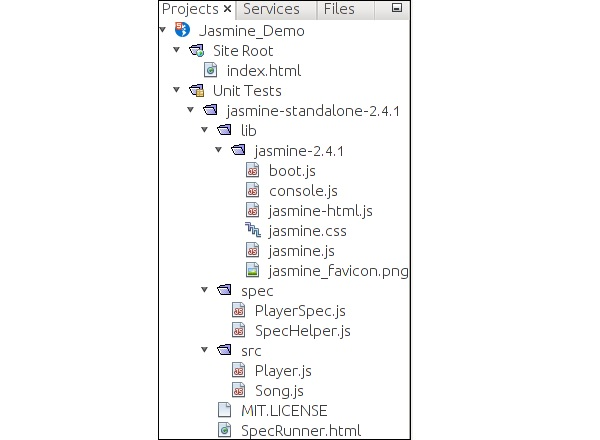Files given under spec and src folders are demo files provided by the Jasmine team. Delete these files as we are going to create our own test file and test case. While deleting those JavaScript file, we need to delete the reference of those files inside our output html file that is SpecRunner.html.

Following is the screenshot of SpecRunner.html file where the reference of different JavaScript files inside spec and src will be deleted.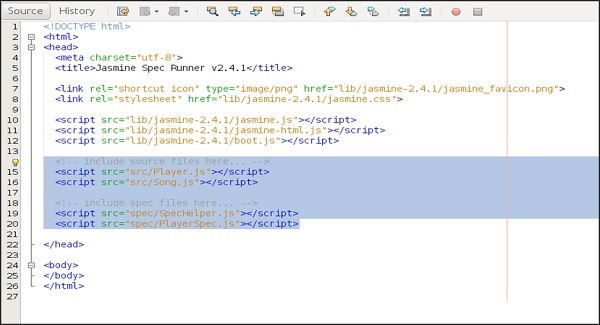Step 3 − Create a JavaScript file

In this step, we will create a JavaScript file named helloworld.js under src folder. This is the file which we will test through Jasmine. After creating a JavaScript file append the following set of code inside the file.

```/*
* This is the JavaScript file that need to be tested through jasmine
* Below is the helloworld function that will return 'Hello World'
*
*/

var helloworld = function() {
return 'Hello World';
};
```

Step 4 − Create a test case

In this step, we will create another JavaScript file which will contain the test case for the above-mentioned JavaScript file. Go ahead and create a JavaScript file under “Spec” folder and name it as “HelloWorldsSpec.js”. Add the following line of code into this js file.

```/*
* This is the file which will call our java script file that need to be tested.
* Each describe block is equivalent to one test case
*
*/

describe("Hello World", function() {

it("should Return Hello world",function() {
expect(helloworld()).toEqual('Hello World');
});

});
```

Step 5 − Add reference to the output file

We successfully created our own file to be tested and the corresponding test case. We kept it under two different folders. In this step, we will modify “SpecRunner.html” to include the reference of these two newly created file.

```<!DOCTYPE html>

<html>
<meta charset = "utf-8">

<title>Jasmine Spec Runner v2.4.1</title>

<link rel = "shortcut icon" type = "image/png" href =
"lib/jasmine2.4.1/jasmine_favicon.png">
<link rel = "stylesheet" href = "lib/jasmine-2.4.1/jasmine.css">

<script src = "lib/jasmine-2.4.1/jasmine.js"></script>
<script src = "lib/jasmine-2.4.1/jasmine-html.js"></script>
<script src = "lib/jasmine-2.4.1/boot.js"></script>

<!--Lines to be deleted
<script src = "src/Player.js"></script>
<script src = "src/Song.js"></script>
<script src = "spec/SpecHelper.js"></script>
<script src = "spec/PlayerSpec.js"></script> -->

<!--adding the reference of our newly created file --->

<script src = "src/helloworld.js"></script>
<script src = "spec/HelloWorldsSpec.js"></script>

<body>
</body>

</html>
```

Step 6 − Execute by running SpecRunner.html

This is the final step of our application development. Run SpecRunner.html in any of your favorite browser. The following screenshot will appear as a result. The green screen indicates success, whereas red indicates failure in test case.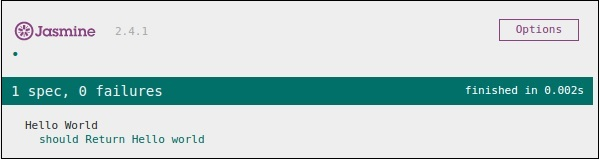Step 7 − Understand the failure case

Till now we have seen the success test case of the hello world application. Now let us see what if something goes wrong and the test fails. To implement a failure case we need to write a failure test case. To do the same, we are going to modify the helloworld.js file using the following code.

```var helloworld = function () {
return '';
};

// we are not returning any string whereas in the spec file
//we are expecting a // string as “Hello World”
```

The above code is definitely going to fail because our spec file is not getting the expected string as an output of the helloworld(). The following screenshot of the specRunner.html file depicts that there is an error with its red indicator.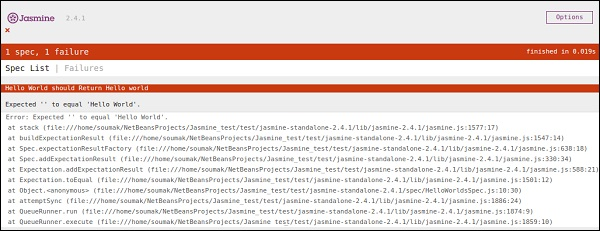# JasmineJS - BDD Architecture

Jasmine follows the Behavioral Driven Development (BDD) framework. Before learning the working principle of Jasmine, let us know what is the BDD framework.

The following flowchart depicts the different phases of BDD framework.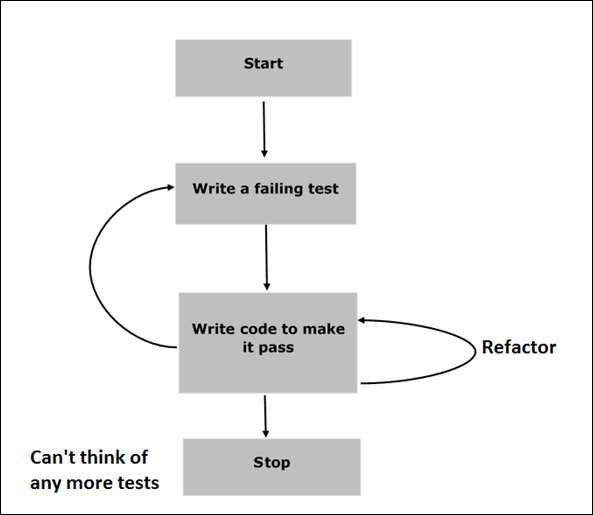Step 1 − Start

In this phase, we will make our environment ready for Jasmine application.

Step 2 − Write a failing test

In this step, we will write our first ever test case. It is obvious that this test is going to fail because there is no such file or function to be tested.

Step 3 − Write a code to make it pass

In this phase, we will prepare our JavaScript file or function that needs to be tested. This phase is crucial as we need to make sure that all the test cases we had prepared in the early stage will be successful.

Step 4 − Refactor

Refactor is a very important phase of BDD model where we need to prepare as many test cases as we can for that particular application or function.

Step 5 − Stop

If everything is going well then your application must be ready and up. So we can consider this step as an end of our BDD application.

## Example

We have now gathered some knowledge about the working principle of BDD framework. Let us see how Jasmine follows this BDD framework in the line of JavaScript testing.

As the screenshot depicts we need to test Abc.js using Jasmine framework. SpecRunner.html is the output file that will take Spec.js(Test case file ), Abc.js(file to be tested), LIB as an input and run all the test cases present in the spec file and render the result into the browser.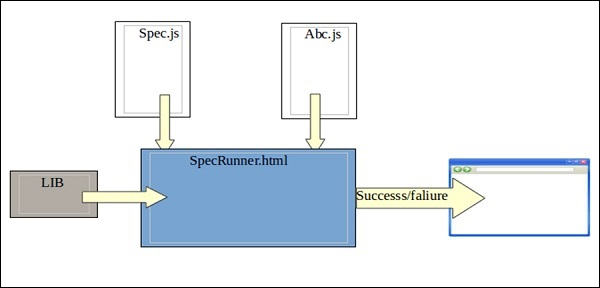Lib − These are the inbuilt JavaScript files that will be helpful to test different functions and other JavaScript files used in our project.

Spec.js(Test case file) − This is the JavaScript file that contains all the test cases which is required to test any JavaScript function or file. In the BDD methodology, we are going to write the test first, hence this is the file that needs to be updated first. Definitely this is going to be fail as there is no such file or function present in our project that can be tested. This file can be refactored unlimited times until all the functionalities are tested.

Abc.js(File to be tested) − This is the file that contains your functionalities which will be unit tested using Spec.js and Lib file.

SpecRunner.html − SpecRunner.html is a normal html file which will render the output of the unit test with the help of embedded JavaScript codes in it.

# JasmineJS - Building Blocks of Test

In this chapter, we will discuss the building blocks of test by Jasmine.

## Suite Block

Jasmine is a testing framework for JavaScript. Suite is the basic building block of Jasmine framework. The collection of similar type test cases written for a specific file or function is known as one suite. It contains two other blocks, one is “Describe()” and another one is “It()”.

One Suite block can have only two parameters, one “name of that suite” and another “Function declaration” that actually makes a call to our unit functionality that is to be tested.

In the following example, we will create a suite that will unit test add function in add.js file. In this example, we have our JS file named “calculator.js” which will be tested through Jasmine, and the corresponding Jasmine spec file is “CalCulatorSpec.js”.

### Calculator.js

```window.Calculator = {

currentVal:0,
varAfterEachExmaple:0,

this.currentVal += num1;
return this.currentVal;
},

var sum = this.currentVal;

for(var i = 0; i < arguments.length; i++) {
sum += arguments[i];
}

this.currentVal = sum;
Return  this.currentVal;
},
};
```

### CalCulatorSpec.js

```describe("calculator",function() {

//test case: 1
it("Should retain the current value of all time", function () {
expect(Calculator.currentVal).toBeDefined();
expect(Calculator.currentVal).toEqual(0);
});

//test case: 2
});

//test case :3
it("Should add any number of numbers",function () {
});
});
```

In the above function, we have declared two functions. Function add will add two numbers given as an argument to that function and another function addAny should add any numbers given as an argument.

After creating this file, we need to add this file in “SpecRunner.html” inside the head section. On successful compilation, this will generate the following output as a result.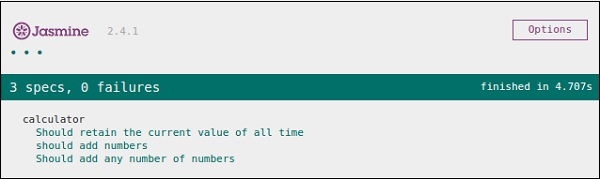## Nested Suites Block

Suite block can have many suite blocks inside another suite block. The following example will show you how we can create a different suite block inside another suite block. We will create two JavaScript files, one named as “NestedSpec.js” and another named as “nested.js”.

### NestedSpec.js

```describe("nested",function() {

// Starting of first suite block
// First block

describe("Retaining values ",function () {

//test case:1
it ("Should retain the current value of all time", function () {
expect(nested.currentVal).toBeDefined();
expect(nested.currentVal).toEqual(0);
});
}); //end of the suite block

//second suite block
describe("Adding single number ",function () {

//test case:2
});
}); //end of the suite block

//third suite block

//test case:3
it("Should add any number of numbers",function() {
});
}); //end of the suite block
});
```

### Nested.js

```window.nested = {

currentVal: 0,

this.currentVal += num1;
return this.currentVal;
},

Var sum = this.currentVal;

for(var i = 0;i < arguments.length; i++) {
sum += arguments[i];
}

this.currentVal = sum;
return this.currentVal;
}
};
```

The above piece of code will generate the following output as a result of running specRunner.html file after adding this file inside the head section.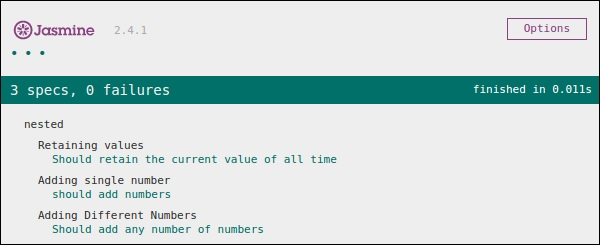## Describe Block

As discussed earlier describe block is a part of Suite block. Like Suite block, it contains two parameters, one “the name of the describe block” and another “function declaration”. In our upcoming examples, we will go through many describe blocks to understand the working flow of Jasmine suite block. Following is an example of a complete describe block.

```describe("Adding single number ",function () {

});
}
```

## IT Block

Like describe block we have been introduced to IT block too. It goes within a describe block. This is the block which actually contains each unit test case. In the following code, there are pieces of IT block inside one describe block.

```describe("Adding single number ",function () {

// test case : 1
});

//test case : 2
});
}
```

## Expect Block

Jasmine Expect allows you to write your expectation from the required function or JavaScript file. It comes under IT block. One IT block can have more than one Expect block.

Following is an example of Expect block. This expect block provides a wide variety of methods to unit test your JavaScript function or JavaScript file. Each of the Expect block is also known as a matcher. There are two different types of matchers, one inbuilt matcher and another user defined matchers.

```describe("Adding single number ",function () {

// test case : 1
});

//test case : 2
});
}
```

In the upcoming chapters, we will discuss various uses of different inbuilt methods of the Expect block.

# JasmineJS - Matchers

Jasmine is a testing framework, hence it always aims to compare the result of the JavaScript file or function with the expected result. Matcher works similarly in Jasmine framework.

Matchers are the JavaScript function that does a Boolean comparison between an actual output and an expected output. There are two type of matchers Inbuilt matcher and Custom matchers.

## Inbuilt Matcher

The matchers which are inbuilt in the Jasmine framework are called inbuilt matcher. The user can easily use it implicitly.

The following example shows how Inbuilt Matcher works in Jasmine framework. We have already used some matchers in the previous chapter.

```describe("Adding single number ", function () {

//example of toEqual() matcher
});

});
}
```

In the example toEqual() is the inbuilt matcher which will compare the result of the add() and addAny() methods with the arguments passed to toEqual() matchers.

## Custom Matchers

The matchers which are not present in the inbuilt system library of Jasmine is called as custom matcher. Custom matcher needs to be defined explicitly(). In the following example, we will see how the custom matcher works.

```describe('This custom matcher example', function() {

beforeEach(function() {
// We should add custom matched in beforeEach() function.
validateAge: function() {
Return {
compare: function(actual,expected) {
var result = {};
result.pass = (actual > = 13 && actual < = 19);
result.message = 'sorry u are not a teen ';
return result;
}
};
}
});
});

it('Lets see whether u are teen or not', function() {
var myAge = 14;
expect(myAge).validateAge();
});

it('Lets see whether u are teen or not ', function() {
var yourAge = 18;
expect(yourAge).validateAge();
});
});
```

In the above example, validateAge() works as a matcher which is actually validating your age with some range. In this example, validateAge() works as a custom matcher. Add this JS file into SpecRunner.html and run the same. It will generate the following output.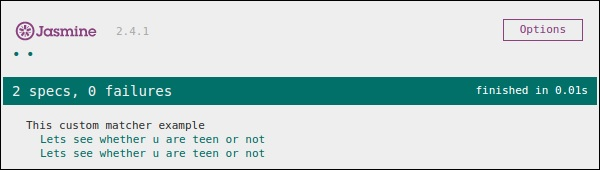# JasmineJS - Skip Block

Jasmine also allows the developers to skip one or more than one test cases. These techniques can be applied at the Spec level or the Suite level. Depending on the level of application, this block can be called as a Skipping Spec and Skipping Suite respectively.

In the following example, we will learn how to skip a specific Spec or Suite using “x” character.

## Skipping Spec

We will modify the previous example using “x” just before it statement.

```describe('This custom matcher example ', function() {

beforeEach(function() {
// We should add custom matched in beforeEach() function.

validateAge: function() {
return {
compare: function(actual,expected) {
var result = {};
result.pass = (actual > = 13 && actual < = 19);
result.message = 'sorry u are not a teen ';
return result;
}
};
}
});
});

it('Lets see whether u are teen or not', function() {
var myAge = 14;
expect(myAge).validateAge();
});

xit('Lets see whether u are teen or not ', function() {
//Skipping this Spec
var yourAge = 18;
});
});
```

If we run this JavaScript code, we will receive the following output as a result in the browser. Jasmine itself will notify the user that the specific it block is disabled temporarily using “xit”.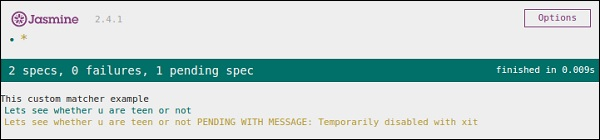## Skipping Suite

In the same way, we can disable the describe block in order to implement the technique of Skipping Suite. In the following example, we will learn about the process of skipping suite block.

```xdescribe('This custom matcher example ', function() {

//Skipping the entire describe  block
beforeEach(function() {

// We should add custom matched in beforeEach() function.
validateAge: function() {
return {
compare: function(actual,expected) {
var result = {};
result.pass = (actual >=13 && actual<=19);
result.message ='sorry u are not a teen ';
return result;
}
};
}
});
});

it('Lets see whether u are teen or not', function() {
var myAge = 14;
expect(myAge).validateAge();
});

it('Lets see whether u are teen or not ', function() {
var yourAge = 18;
expect(yourAge).validateAge();
});
});
```

The above code will generate the following screenshot as an output.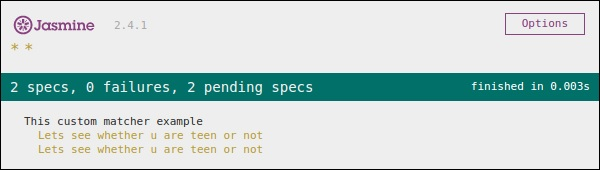As we can see in the message bar, it shows two spec blocks in pending status, which means these two Spec blocks is disabled using “x” character. In the upcoming chapter, we will discuss different types of Jasmine test scenarios.

# JasmineJS - Equality Check

Jasmine provides plenty of methods which help us check the equality of any JavaScript function and file. Following are some examples to check equality conditions.

## ToEqual()

ToEqual() is the simplest matcher present in the inbuilt library of Jasmine. It just matches whether the result of the operation given as an argument to this method matches with the result of it or not.

The following example will help you understand how this matcher works. We have two files to be tested named as “expectexam.js” and another one through which we need to test is “expectSpec.js”.

### Expectexam.js

```window.expectexam = {
currentVal: 0,
};
```

### ExpectSpec.js

```describe("Different Methods of Expect Block",function () {

it("The Example of toEqual() method",function () {
//this will check whether the value of the variable
// currentVal is equal to 0 or not.
expect(expectexam.currentVal).toEqual(0);
});
});
```

On successful execution, these pieces of code will yield the following output. Remember you need to add these files into the header section of specRunner.html file as directed in the earlier example.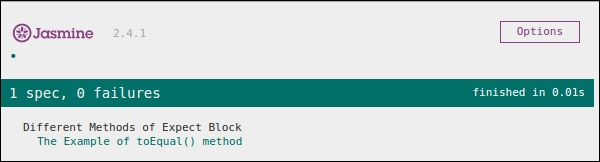## not.toEqual()

not.toEqual() works exactly opposite to toEqual(). not.toEqual() is used when we need to check if the value does not match with the output of any function.

We will modify the above example to show how this works.

### ExpectSpec.js

```describe("Different Methods of Expect Block",function () {

it("The Example of toEqual() method",function () {
expect(expectexam.currentVal).toEqual(0);
});

it("The Example of not.toEqual() method",function () {
//negation  testing expect(expectexam.currentVal).not.toEqual(5);
});
});
```

### Expectexam.js

```window.expectexam = {
currentVal: 0,
};
```

In the second expect block, we are checking whether the value of the currentVal is equal to 5 as the value of currentVal is zero hence our test passes and provides us with a green output.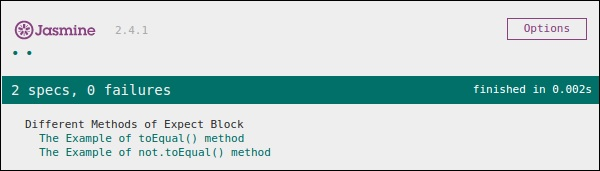## ToBe()

toBe() matcher works in a similar way as toEqual(), however they are technically different from each other. toBe() matcher matches with the type of the object whereas toEqual() matches with the equivalency of the result.

The following example will help you understand the working principle of the toBe() matcher. This matcher is exactly equivalent to the “===” operator of JavaScript whereas toEqual() is similar to the “==” operator of JavaScript.

### ExpectSpec.js

```describe("Different Methods of Expect Block",function () {

it("The Example of toBe() method",function () {
expect(expectexam.name).toBe(expectexam.name1);
});
});
```

### Expectexam.js

```window.expectexam = {
currentVal: 0,
name:"tutorialspoint",
name1:tutorialspoint
};
```

We will slightly modify our expectexam JavaScript file. We added two new variables, name and name1. Please find the difference between these two added variables - one is of string type and another one is not a string type.

Following screenshot is our test result where the red cross depicts that these two values are not equal, whereas it is expected to be equal. Hence our test fails.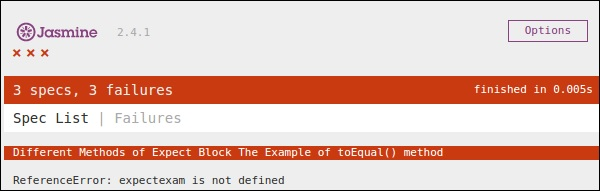Let us turn both the variables, name and name1 as String type variables and run the same SpecRunner.html again. Now check the output. It will prove that toBe() not only matches with the equivalency of the variable, but it also matches with the data type or object type of the variable.

## not.toBe()

As seen earlier, not is nothing but a negation of the toBe() method. It fails when the expected result matches with the actual output of the function or JavaScript file.

Following is a simple example that will help you understand how not.toBe() matcher works.

```describe("Different Methods of Expect Block",function () {
it("The Example of not.toBe() method",function () {
expect(true).not.toBe(false);
});
});
```

Here Jasmine will try to match up true with false. As true cannot be same as false, this test case will be valid and pass through.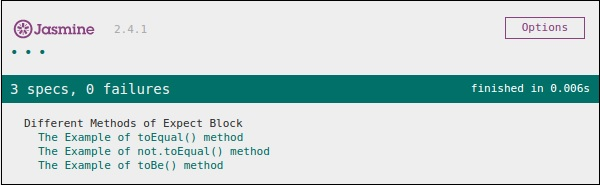# JasmineJS - Boolean Check

Apart from equality check, Jasmine provides some methods to check Boolean conditions too. Following are the methods that help us check Boolean conditions.

## ToBeTruthy()

This Boolean matcher is used in Jasmine to check whether the result is equal to true or false.

The following example will help us understand the working principle of the toBeTruthy() function.

### ExpectSpec.js

```describe("Different Methods of Expect Block",function () {
it("The Example of toBeTruthy() method",function () {
expect(expectexam.exampleoftrueFalse(5)).toBeTruthy();
});
});
```

### Expectexam.js

```window.expectexam = {
exampleoftrueFalse: function (num) {
if(num < 10)
return true;
else
return false;
},
};
```

As we are passing number 5, which is smaller than 10, this test case will pass and give us the following output.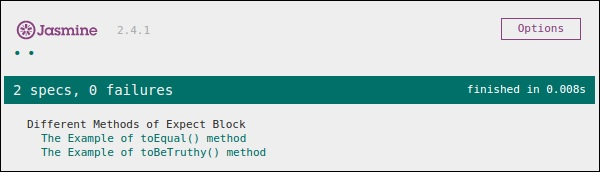If we pass a number which is larger than 10, then this green test will change to red. In the second screenshot, you can see that on passing some value which is greater than 10, the expected test case fails and generates red output stating that “Expected false to be truthy”.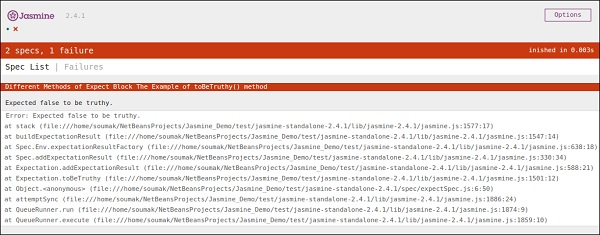## toBeFalsy()

toBeFalsy() also works the same way as toBeTruthy() method. It matches the output to be false whereas toBeTruthy matches the output to be true. The following example will help you understand the basic working principles of toBeFalsy().

### ExpectSpec.js

```describe("Different Methods of Expect Block",function() {
it("The Example of toBeTruthy() method",function () {
expect(expectexam.exampleoftrueFalse(15)).toBeFalsy();
});
});
```

### Expectexam.js

```window.expectexam = {
exampleoftrueFalse: function (num) {
if(num < 10)
Return true;
else
return false;
},
};
```

The above code will pass the Jasmine test case as we are passing value more than 10 and expected the output to be false. Hence, the browser will show us a green sign which means it has passed.# JasmineJS - Sequential Check

Jasmine also provides different methods to provide sequentiality of the JS output. Following examples show how to implement sequential check using Jasmine.

## ToContain()

toContain() matchers provide us the facility to check whether any element is a part of the same array or some other sequential objects. The following example will help us understand the working methodology of Jasmine toContain() method. Let's add the following piece of code in previously created customerMatcherSpec.js file.

```describe("Different Methods of Expect Block",function () {
it("The  Example of toContain() method",function () {
expect([1,2, 3, 4]).toContain(3);
});
});
```

In the above example, we are checking whether 3 is present in that array or not. We get a green output as 3 is present in the array.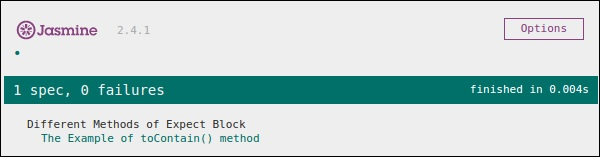In the above example, let's change the value of 3 with 15 and run the spec again. We will get the following red screen as 15 does not belong to that array we are passing as a parameter of that function.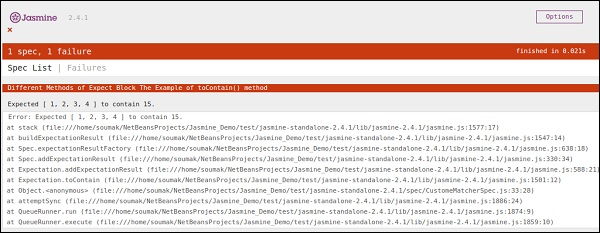## ToBeCloseTo()

toBeCloseTo() matcher matches whether the actual value is close to the expected value. In the following example, we will modify our customerMatcherSpec.js file and see how this actually works.

```describe("Different Methods of Expect Block", function () {
it("Example of toBeCloseTo()", function () {
expect(12.34).toBeCloseTo(12.3, 1);
});
});
```

In the above Describe block, we are checking whether the actual result “12.3” is closer to the expected output “12.34” or not. As this satisfies our requirement, we will have the following green screenshot as our output. The second parameter of this method is the count of the decimal place to be compared with.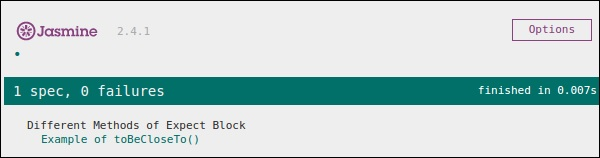In the above code, let's modify the expected value to 15 and run SpecRunner.html.

```describe("Different Methods of Expect Block",function () {
it("Example of  toBeCloseTo()", function () {
expect(12.34).toBeCloseTo(15, 1);
});
});
```

In this scenario, 15 is nowhere close to 15, hence it will generate an error and present a red screenshot as an error.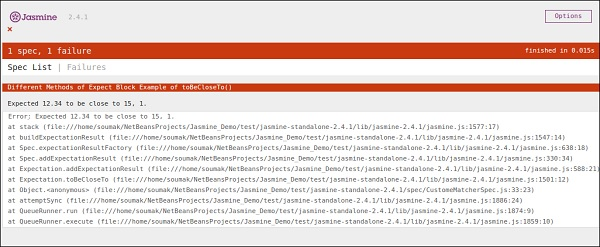## ToMatch()

ToMatch() matcher works on String type variable. It is helpful to find whether a specific String is present in the expected output or not. Following is what our customerMatcherSpec.js looks like.

```describe("Different Methods of Expect Block",function () {
it("Example of toMatch()", function () {
expect("Jasmine tutorial in tutorials.com").toMatch(/com/);
});
});
```

This piece of code will test whether “com” is present in the expected String given. As com exists in the string, it will generate a green screenshot and pass the test condition.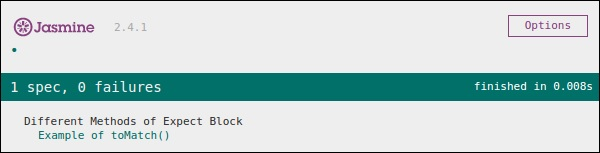Now let us change the output to some other string, which is not present in the expected value. Then our customerMatcherSpec.js will look like the following.

```describe("Different Methods  of Expect Block",function () {
it("Example of toMatch()", function () {
expect("Jasmine tutorial in tutorials.com").toMatch(/XYZ/);
});
});
```

The above code will find “XYZ” string in the expected value. As it does not exist in the expected string, it will throw an error and the output screen will be red accordingly.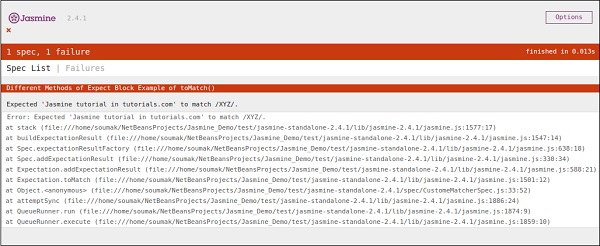# JasmineJS - Null Check

Jasmine provides a different variety of method to check whether the actual output is Null, defined or undefined. In this chapter, we will learn how to implement different Jasmine methods to check the above-mentioned scenarios.

## ToBedefined()

This matcher is used to check whether any variable in the code is predefined or not. Let us modify our customerMatcherSpec.js file according to this example.

```currentVal = 0;

describe("Different Methods  of Expect Block",function () {
it("Example of  toBeDefined", function () {
expect(currentVal).toBeDefined();
});
});
```

In the above code, toBeDefined() will check whether the variable currentVal is defined in the system or not. As currentVal is defined to 0 in the beginning, this test will pass and generate a green screenshot as an output.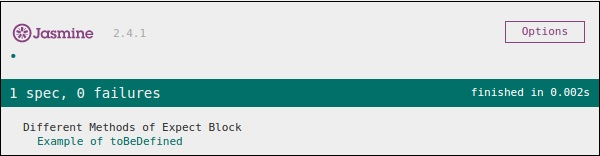Again in the above example, let us remove the first line, where we actually define “currentVal” and run again. Then we will get a red screen, which means the test actually fails because we are expecting an undefined value to be defined. The following screenshot will be the output file.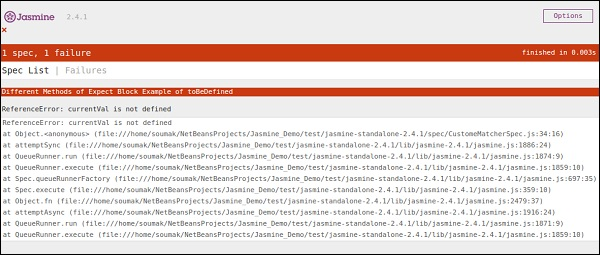## ToBeUndefined()

This matcher helps to check whether any variable is previously undefined or not, basically it works simply opposite to the previous matcher that is toBeDefined. In the following example, we will learn how to use this matcher. Let us modify our Spec file, i.e. customerMatcher.js file with the following entry.

```describe("Different Methods of Expect Block",function () {
it("Example of toBeUndefine()", function () {
var undefineValue;
expect(undefineValue).toBeUndefined();
});
});
```

In the above section, we will verify whether our variable “undefineValue” is actually undefined or not. After adding this file into the SpecRunner, we will receive a green color screenshot as an output, which tells us that this value is actually not defined previously.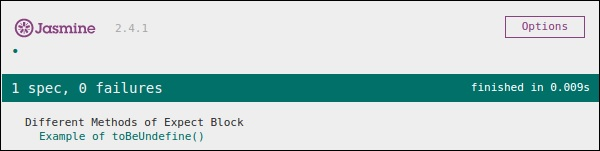Again let us define the variable with some predefined value and see whether it will throw an error or not. The new customerMatcher.js looks like the following.

```describe("Different Methods of Expect Block",function () {
it("Example oftoBeUndefine()", function () {
var undefineValue = 0;
expect(undefineValue).toBeUndefined();
});
});
```

The above piece of code will throw an error and generate a red color screenshot because we have already defined the “undefineValue” value to “0” and expecting it to be not defined. The following screenshot will be generated on run SpecRunner.html file.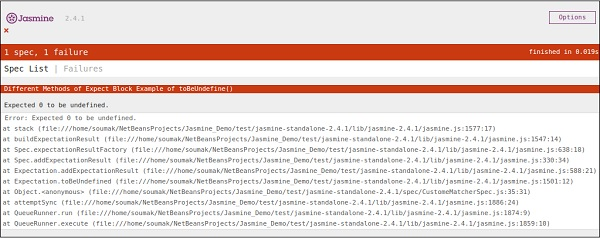## toBeNull()

As the name signifies this matcher helps to check null values. Let us again modify our customerMatcherSpec.js file with the following piece of code.

```describe("Different Methods of Expect Block",function () {
var value = null;

it("Example of toBeNull()", function () {
expect(value).toBeNull();
});
});
```

In the above code, we have mentioned one variable ”value” and we have explicitly mentioned this value as null. In the expect block, the toBeNull() matcher will check this value and give us the result accordingly. Following is the output of the above-mentioned code when it is run through the help of the SpecRunner.html file.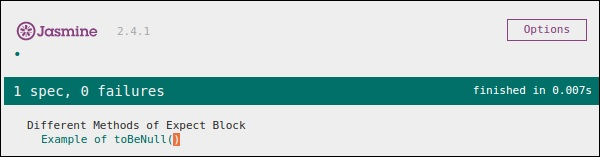Now let us test by providing some defined value other than null. Please modify the customerMatcher.js file accordingly.

```describe("Different Methods of Expect Block",function () {
var value = "TutorialsPoint";

it("Example of  toBeNull()", function () {
expect(value).toBeNull();
});
});
```

In the above example, we have modified the variable value with “TutorialsPoint” which is not a null value. Hence, this test will fail and produce a red screenshot as an output.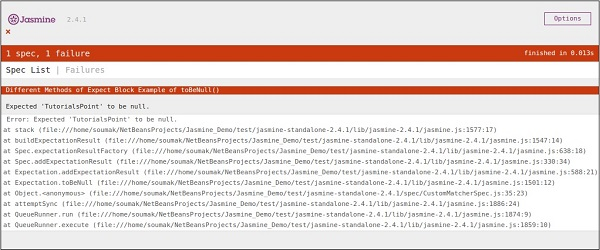# JasmineJS - Inequality Check

Till now, we have discussed different methods in Jasmine which help us test different scenarios based on our requirements. In this chapter, we will learn about different matchers that will help us check the inequality condition in JS file. Following are the matchers used for this purpose.

## ToBeGreaterThan()

As the name suggests this matcher helps to check greater than condition. Let us modify our customerMatcher.js using the following piece of code.

```describe("Different Methods of Expect Block",function () {
var exp = 8;

it("Example of  toBeGreaterThan()", function () {
expect(exp).toBeGreaterThan(5);
});
});
```

In the above piece of code, we are expecting that the value of the variable “exp” will be greater than 5. Now as the value of the variable “exp” is “8” which is greater than “5”, this piece of code will generate a green screenshot.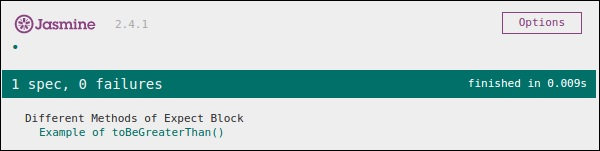Now again let us modify the value of the variable to “4” and make this test fail. To do that we need to modify the js file using the following piece of code.

```describe("Different Methods of Expect Block",function () {
var exp = 4;

it ("Example of toBeGreaterThan()", function () {
expect(exp).toBeGreaterThan(5);
});
});
```

This code will fail because value 4 cannot be greater than 5. Hence it will produce the following output.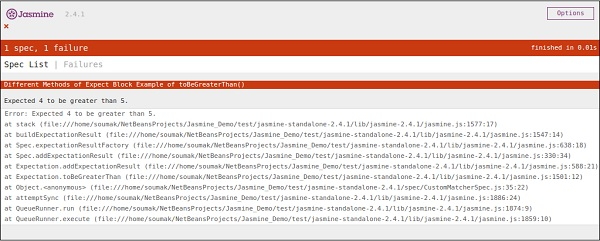## ToBeLessThan()

This matcher helps to check the less than condition of the test scenario. It behaves exactly opposite to that of toBeGreaterThan() matcher. Now let us see how this matcher works. Let us modify the customerMatcher.js file accordingly.

```describe("Different Methodsof Expect Block",function () {
var exp = 4;

it("Example of toBeLessThan()", function() {
expect(exp).toBeLessThan(5);
});
});
```

Like the previous example, we have one variable having value as “4”. In this piece of code, we are checking whether the value of this variable is less than 5 or not. This piece of code will generate the following output.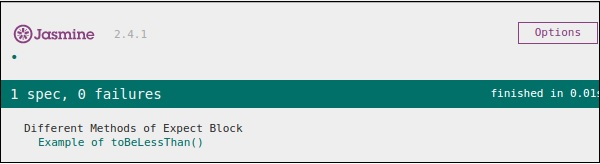Now to make this fail, we need to assign some bigger number to the variable exp. Let us do that and test the application. We will assign 25 as the value to the exp, which will definitely throw an error and yield the following screenshot in red.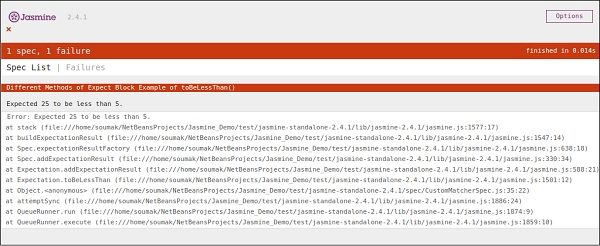# JasmineJS - Not a Number Check

Jasmine provides a special matcher to check this special type of testing scenario that is toBeNaN().

Let us modify our customerMatcher.js with the following code.

```describe("Different Methods of Expect Block",function () {
it("Example of toBeNaN()", function () {
expect(0 / 0).toBeNaN();
});
});
```

Here we want to test what is the value of “0/0” which cannot be determined. Hence, this piece of code will generate the following green screenshot.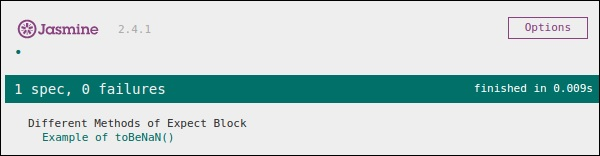Now let us again modify the code with the following logic, where we will assign one variable exp to 25 and expect the result is not a number one dividing it with 5.

```describe("Different Methods of Expect Block",function () {
var exp = 25;

it("Example of toBeNaN()", function () {
expect(exp/5).toBeNaN();
});
});
```

This piece of code will yield the following output.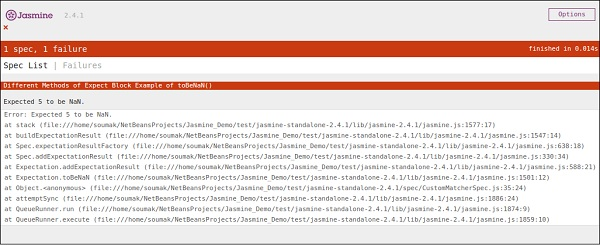# JasmineJS - Exception Check

Apart from different computational matchers, Jasmine provides some useful matchers to check exception of the program. Let us modify our JavaScript with the following set of code.

```var throwMeAnError = function() {
throw new Error();
};

describe("Different Methods of Expect Block", function() {
var exp = 25;

it ("Hey this will throw an Error ", function() {
expect(throwMeAnError).toThrow();
});
});
```

In the above example, we have created one method which deliberately throws an exception from that method and in the expect block we expect to catch the error. If everything goes well then this piece of code will yield the following output.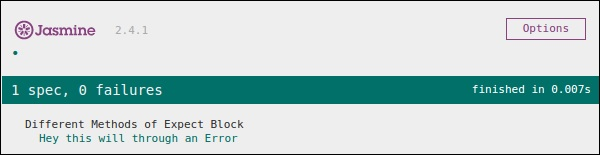Now, for this test case to fail, we need to omit that throw statement in the function throwMeAnError. Following is the code which will yield a red screenshot as an output since the code does not satisfy our requirement.

```var throwMeAnError = function() {
//throw new Error();
};

describe("Different Methods of Expect Block",function() {
var exp = 25;

it("Hey this will throw an Error ", function() {
expect(throwMeAnError).toThrow();
});
});
```

As can be seen, we have commented that line from where our method was throwing the exception. Following is the output of the above code on successful execution of the SpecRunner.html.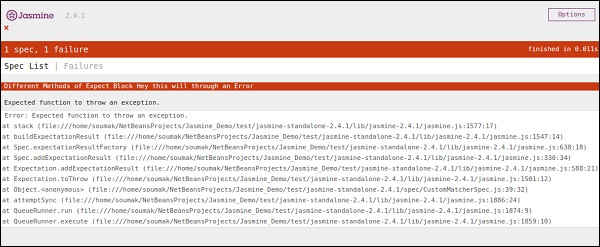## Jasmine.Any()

Any is the special matcher that is used when we are not sure about the output. In the following example, we will learn how this works. Let us modify the customerMatcher.js with the following piece of code.

```var addAny = function() {
var sum = this.currentVal;

for (var i = 0; i < arguments.length; i++) {
sum += arguments[i];
}

this.currentVal = sum;
return this.currentVal;
}

describe("Different Methods of Expect Block",function () {

it("Example of any()", function() {
});
});
```

Here we have declared one function that will give us the summation of the numbers provided as arguments. In the expect block, we are expecting that the result can be anything but it should be a Number.

As both 9 and 9 after sum yield 18 is a number, this test will pass and it will generate the following green screenshot as an output.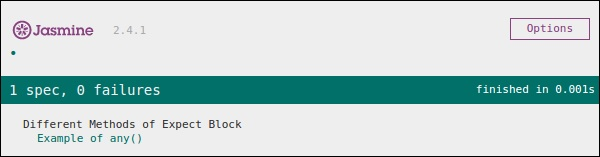Now let us change the code according to the following piece of code, where we are expecting a string type variable as an output of the function AddAny().

```var addAny = function() {
var sum = this.currentVal;

for(var i = 0; i < arguments.length; i++) {
sum += arguments[i];
}

this.currentVal = sum;
return this.currentVal;
}

describe("Different Methodsof Expect Block",function () {
it("Example of any()", function () {
});
});
```

Following is the output of the above code.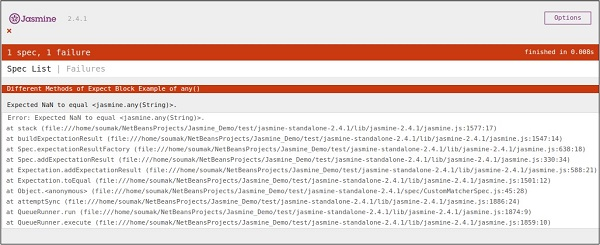# JasmineJS - beforeEach()

Another notable feature of Jasmine is before and after each function. Using these two functionalities, we can execute some pieces of code before and after execution of each spec. This functionality is very useful for running the common code in the application. Let us create one spec file like the following.

```var currentVal = 0;

beforeEach(function() {
currentVal = 5;
});

describe("Different Methods of Expect Block",function() {
it("after each function ", function() {
expect(currentVal).toEqual(5);
});
});
```

Here although we have declared one variable as “0” in the beginning, we are expecting this value should be equal to 5 in the expectation block. The above code will generate the following output.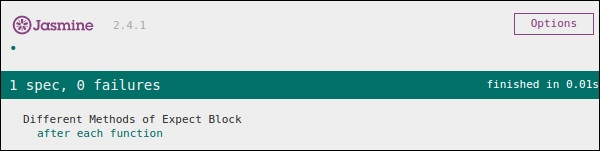In the above code, 5 will be assigned to a variable currentVal before the execution of the expect block. Hence, it generates a green screenshot with no error.

# JasmineJS - afterEach()

Like beforeEach(), afterEach() works exactly the same way. It executes after the execution of the spec block. Let us modify the previous example using the following code.

```var currentVal = 0;

afterEach(function() {
currentVal = 5;
});

describe("Different Methods of Expect Block",function() {
it("first call ", function() {
expect(currentVal).toEqual(0);
});

it("second call ",  function() {
expect(currentVal).toEqual(5);
});
});
```

In the above example, while running the first spec block the value of the currentVal is 0. Hence, it will pass the test case but after running the first it block, Jasmine compile ran the afterEach() block, which makes the value of the currentVal to 5. Hence it also satisfies the second case and yields a green screenshot as an output.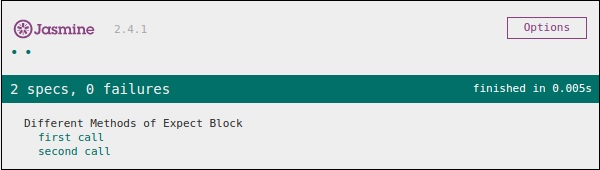# JasmineJS - Spies

Jasmine spy is another functionality which does the exact same as its name specifies. It will allow you to spy on your application function calls. There are two types of spying technology available in Jasmine. The first methodology can be implemented by using spyOn() and the second methodology can be implemented using createSpy(). In this chapter, we will learn more about these two methodologies.

## spyOn()

spyOn() is inbuilt into the Jasmine library which allows you to spy on a definite piece of code. Let us create a new spec file “spyJasmineSpec.js” and another js file named as “spyJasmine.js”. Following is the entry of these two files.

### SpyJasmine.js

```var Person = function() {};

Person.prototype.sayHelloWorld = function(dict) {
return dict.hello() + " " + dict.world();
};

var Dictionary = function() {};

Dictionary.prototype.hello = function() {
return "hello";
};

Dictionary.prototype.world = function() {
return "world";
};
```

### SpyJasmineSpec.js

```describe("Example Of jasmine Spy using spyOn()", function() {

it('uses the dictionary to say "hello world"', function() {
var dictionary = new Dictionary;
var person = new Person;

spyOn(dictionary, "hello");  // replace hello function with a spy
spyOn(dictionary, "world");  // replace world function with another spy

person.sayHelloWorld(dictionary);
expect(dictionary.hello).toHaveBeenCalled();
// not possible without first spy

expect(dictionary.world).toHaveBeenCalled();
// not possible withoutsecond spy
});
});
```

In the above piece of code, we want person object to say “Hello world” but we also want that person object should consult with dictionary object to give us the output literal “Hello world”.

Take a look at the Spec file where you can see that we have used spyOn() function, which actually mimics the functionality of the hello and world function. Hence, we are not actually calling the function but mimicking the function call. That is the specialty of Spies. The above piece of code will yield the following output.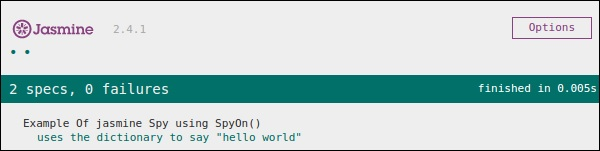## createSpy()

Another method of obtaining the spying functionality is using createSpy(). Let us modify our two js files using the following code.

### SpyJasmine.js

```var Person = function() {};

Person.prototype.sayHelloWorld = function(dict) {
return dict.hello() + " " + dict.world();
};

var Dictionary = function() {};

Dictionary.prototype.hello = function() {
return "hello";
};

Dictionary.prototype.world = function() {
return "world";
};
```

### SpyJasmineSpec.js

```describe("Example Of jasmine Spy using Create Spy", function() {

it("can have a spy function", function() {
var person = new Person();
person.getName11 = jasmine.createSpy("Name spy");
person.getName11();
expect(person.getName11).toHaveBeenCalled();
});
});
```

Take a look at the spec file, we are calling the getName11() of the Person object. Although this function is not present in the person object in spy Jasmine.js, we are not getting any error and hence the output is green and positive. In this example, createSpy() method actually mimics the functionality of the getName11().

The above code will generate the following output.